Lec 2 - Span

Friday, January 06, 2012

9:27 AM

Recall: A set of vectors is closed under linear combinations if we can take a linear combination of vectors in the set and obtain a vector in the set.

Eg.Eg.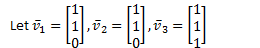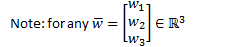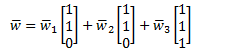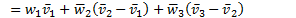Eg.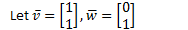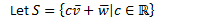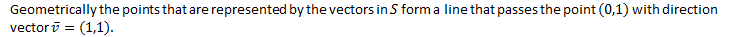Note: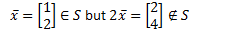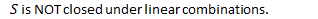In general: Sets of vectors that are closed under linear combinations require only a small number of vectors to describe the entire set.

Definition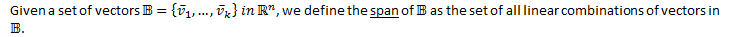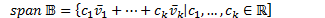Eg.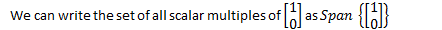Eg.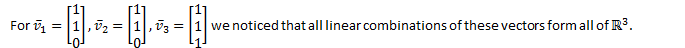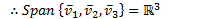Eg.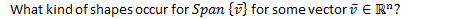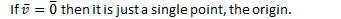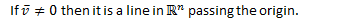Eg.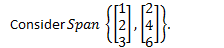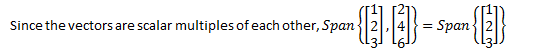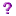How do we find the smallest # of vectors needed in a spanning set?

TheoremProof: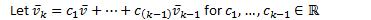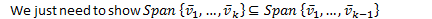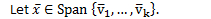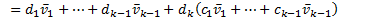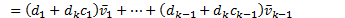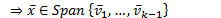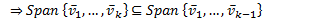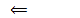Q.E.D.

Eg.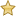The vector equation of the plan isNote: To find a smallest spanning set, we need to identify when one vector the set is a linear combination of the remaining vectors in the set.Linear Dependence

Definition

A set of vectors is linearly dependent if one vector in the set is a linear combination of the remaining vectors.

Otherwise, the set of vectors is linearly independent.

Eg.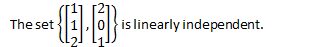Created by Tim Pei with Microsoft OneNote 2010
One place for all your notes and information